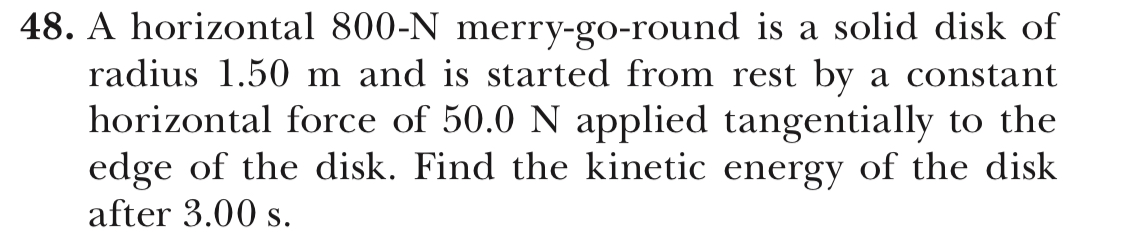# 48. A horizontal 800-N merry-go-round is a solid disk ofradius 1.50 m and is started from rest by a constanthorizontal force of 50.0 N applied tangentially to theedge of the disk. Find the kinetic energy of the diskafter 3.00 s.

Question
74 viewshelp_outlineImage Transcriptionclose48. A horizontal 800-N merry-go-round is a solid disk of radius 1.50 m and is started from rest by a constant horizontal force of 50.0 N applied tangentially to the edge of the disk. Find the kinetic energy of the disk after 3.00 s. fullscreen
check_circle

Step 1

Equations for the moment of inertia of disc about an axis passing through the centre and perpendicular to plane of disc and mass from weight are,

Step 2

Calculate I using the first equation.

Step 3

Calculate I using the fir...

### Want to see the full answer?

See Solution

#### Want to see this answer and more?

Solutions are written by subject experts who are available 24/7. Questions are typically answered within 1 hour.*

See Solution
*Response times may vary by subject and question.
Tagged in

### Physics